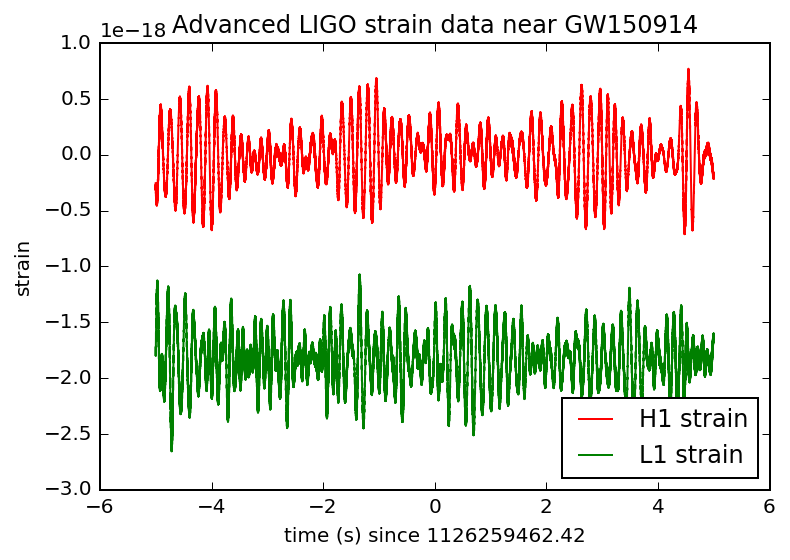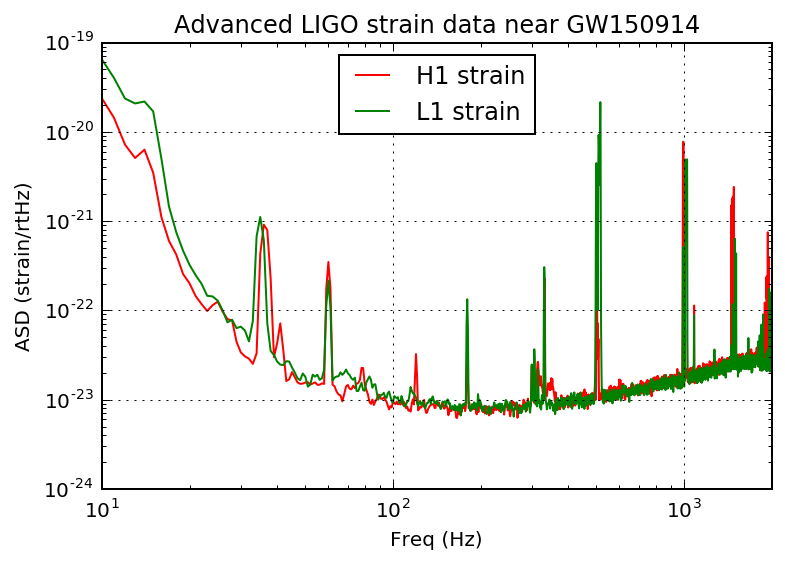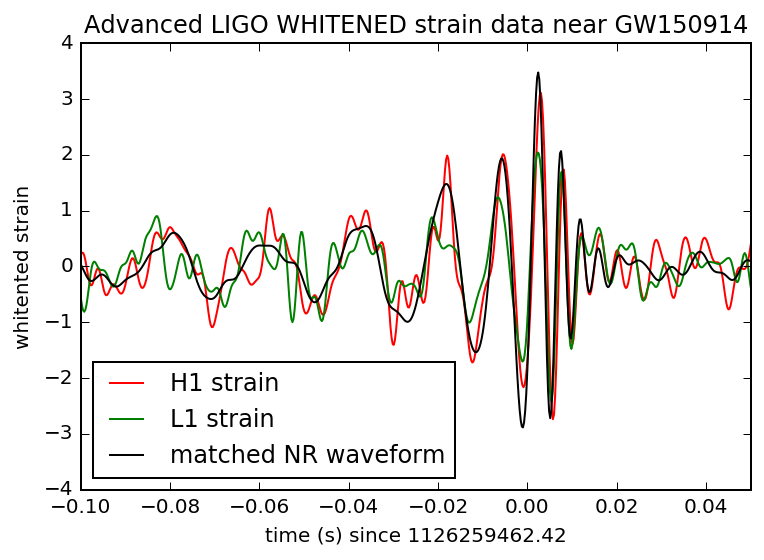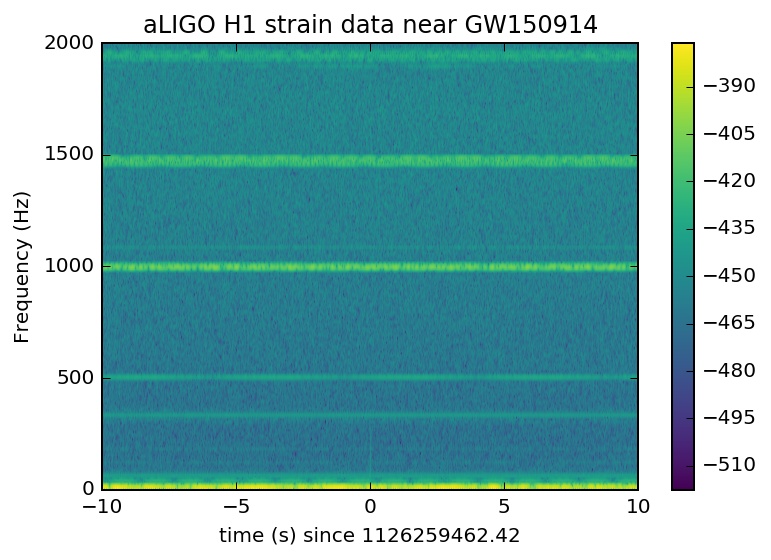CoCalc Public Filessupport / 2016-02-12-LIGO / GW150914_tutorial.html
Description: Jupyter html version of support/2016-02-12-LIGO/GW150914_tutorial.ipynb
Compute Environment: Ubuntu 18.04 (Deprecated)
(File too big to render with math typesetting.)
GW150914_tutorial

To use in SageMathCloud: make an account and new project, then click "Files", "Check all", "Copy" and select the destination project. Everything should work.

# SIGNAL PROCESSING WITH GW150914 OPEN DATA¶

Welcome! This ipython notebook (or associated python script GW150914_tutorial.py ) will go through some typical signal processing tasks on strain time-series data associated with the LIGO GW150914 data release from the LIGO Open Science Center (LOSC):

To begin, download the ipython notebook, readligo.py, and the data files listed below, into a directory / folder, then run it. Or you can run the python script GW150914_tutorial.py. You will need the python packages: numpy, scipy, matplotlib, h5py.

On Windows, or if you prefer, you can use a python development environment such as Anaconda (https://www.continuum.io/why-anaconda) or Enthought Canopy (https://www.enthought.com/products/canopy/).

Questions, comments, suggestions, corrections, etc: email [email protected]

v20160208b

## Intro to signal processing¶

This tutorial assumes that you know python well enough.

If you know how to use "ipython notebook", use the GW150914_tutorial.ipynb file. Else, you can use the GW150914_tutorial.py script.

This tutorial assumes that you know a bit about signal processing of digital time series data (or want to learn!). This includes power spectral densities, spectrograms, digital filtering, whitening, audio manipulation. This is a vast and complex set of topics, but we will cover many of the basics in this tutorial.

If you are a beginner, here are some resources from the web:

And, well, lots more - google it!

• We will use the hdf5 files, both H1 and L1, with durations of 32 and 4096 seconds around GW150914, sampled at 16384 and 4096 Hz :
• From a unix/mac-osx command line, you can use wget; for example,
• Put these files in your current directory / folder. Don't mix any other LOSC data files in this directory, or readligo.py may get confused.

Here,

• "H-H1" means that the data come from the LIGO Hanford Observatory site and the LIGO "H1" datector;
• the "4" means the strain time-series data are (down-)sampled from 16384 Hz to 4096 Hz;
• the "V1" means version 1 of this data release;
• "1126257414-4096" means the data starts at GPS time 1126257414 (Mon Sep 14 09:16:37 GMT 2015), duration 4096 seconds;
• the filetype "hdf5" means the data are in hdf5 format: https://www.hdfgroup.org/HDF5/

Note that the the 4096 second long files at 16384 Hz sampling rate are fairly big files (125 MB). You won't need them for this tutorial:

In :
# Standard python numerical analysis imports:
import numpy as np
from scipy import signal
from scipy.interpolate import interp1d
from scipy.signal import butter, filtfilt, iirdesign, zpk2tf, freqz

# the ipython magic below must be commented out in the .py file, since it doesn't work.
%matplotlib inline
%config InlineBackend.figure_format = 'retina'
import matplotlib.pyplot as plt
import matplotlib.mlab as mlab
import h5py



NOTE that in general, LIGO strain time series data has gaps (filled with NaNs) when the detectors are not taking valid ("science quality") data. Analyzing these data requires the user to loop over "segments" of valid data stretches. In https://losc.ligo.org/segments/ we provide example code to do this.

However, the 4096 seconds of released data around GW150914 is one unbroken segment, with no gaps. So for now, we will read it all in and treat it as one valid data segment, ignoring the extra complexity mentioned above.

This won't work for other LOSC data releases! See https://losc.ligo.org/segments/ for a more general way to find valid data segments in LOSC data.

In :
#----------------------------------------------------------------
# Load LIGO data from a single file
#----------------------------------------------------------------
# First from H1
fn_H1 = 'H-H1_LOSC_4_V1-1126259446-32.hdf5'
strain_H1, time_H1, chan_dict_H1 = rl.loaddata(fn_H1, 'H1')
# and then from L1
fn_L1 = 'L-L1_LOSC_4_V1-1126259446-32.hdf5'
strain_L1, time_L1, chan_dict_L1 = rl.loaddata(fn_L1, 'L1')

# sampling rate:
fs = 4096
# both H1 and L1 will have the same time vector, so:
time = time_H1
# the time sample interval (uniformly sampled!)
dt = time - time


## Adding a numerical relativity template¶

Now let's also read in a theoretical (numerical relativity) template, generated with parameters favored by the output from the GW150914 parameter estimation (see the GW150914 detection paper, https://dcc.ligo.org/P150914/public ).

This NR template corresponds to the signal expected from a pair of black holes with masses of around 36 and 29 solar masses, merging into a single black hole of 62 solar masses, at a distance of around 410 Mpc.

You can fetch the template time series from the following URL, and put it in your working directory / folder:

In :
# read in the NR template
NRtime, NR_H1 = np.genfromtxt('GW150914_4_NR_waveform.txt').transpose()


## First look at the data from H1 and L1¶

In :
# First, let's look at the data and print out some stuff:

# this doesn't seem to work for scientific notation:
# np.set_printoptions(precision=4)

print '  time_H1: len, min, mean, max = ', \
len(time_H1), time_H1.min(), time_H1.mean(), time_H1.max()
print 'strain_H1: len, min, mean, max = ', \
len(strain_H1), strain_H1.min(),strain_H1.mean(),strain_H1.max()
print 'strain_L1: len, min, mean, max = ', \
len(strain_L1), strain_L1.min(),strain_L1.mean(),strain_L1.max()

#What's in chan_dict? See https://losc.ligo.org/archive/dataset/GW150914/
bits = chan_dict_H1['DATA']
print 'H1     DATA: len, min, mean, max = ', len(bits), bits.min(),bits.mean(),bits.max()
bits = chan_dict_H1['CBC_CAT1']
print 'H1 CBC_CAT1: len, min, mean, max = ', len(bits), bits.min(),bits.mean(),bits.max()
bits = chan_dict_H1['CBC_CAT2']
print 'H1 CBC_CAT2: len, min, mean, max = ', len(bits), bits.min(),bits.mean(),bits.max()
bits = chan_dict_L1['DATA']
print 'L1     DATA: len, min, mean, max = ', len(bits), bits.min(),bits.mean(),bits.max()
bits = chan_dict_L1['CBC_CAT1']
print 'L1 CBC_CAT1: len, min, mean, max = ', len(bits), bits.min(),bits.mean(),bits.max()
bits = chan_dict_L1['CBC_CAT2']
print 'L1 CBC_CAT2: len, min, mean, max = ', len(bits), bits.min(),bits.mean(),bits.max()
print 'In both H1 and L1, all 32 seconds of data are present (DATA=1), '
print "and all pass data quality (CBC_CAT1=1 and CBC_CAT2=1)."

  time_H1: len, min, mean, max =  131072 1126259446.0 1126259462.0 1126259478.0
strain_H1: len, min, mean, max =  131072 -7.11996338709e-19 8.73279794057e-23 7.71483633765e-19
strain_L1: len, min, mean, max =  131072 -2.6788089173e-18 -1.82870749189e-18 -7.69266177024e-19
H1     DATA: len, min, mean, max =  32 1 1.0 1
H1 CBC_CAT1: len, min, mean, max =  32 1 1.0 1
H1 CBC_CAT2: len, min, mean, max =  32 1 1.0 1
L1     DATA: len, min, mean, max =  32 1 1.0 1
L1 CBC_CAT1: len, min, mean, max =  32 1 1.0 1
L1 CBC_CAT2: len, min, mean, max =  32 1 1.0 1
In both H1 and L1, all 32 seconds of data are present (DATA=1),
and all pass data quality (CBC_CAT1=1 and CBC_CAT2=1).

In :
# plot +- 5 seconds around the event:
tevent = 1126259462.422         # Mon Sep 14 09:50:45 GMT 2015
deltat = 5.                     # seconds around the event
# index into the strain time series for this time interval:
indxt = np.where((time_H1 >= tevent-deltat) & (time_H1 < tevent+deltat))

plt.figure()
plt.plot(time_H1[indxt]-tevent,strain_H1[indxt],'r',label='H1 strain')
plt.plot(time_L1[indxt]-tevent,strain_L1[indxt],'g',label='L1 strain')
plt.xlabel('time (s) since '+str(tevent))
plt.ylabel('strain')
plt.legend(loc='lower right')
plt.title('Advanced LIGO strain data near GW150914')
plt.savefig('GW150914_strain.png')The data are dominated by low frequency noise; there is no way to see a signal here, without some signal processing.

There are very low frequency oscillations that are putting the mean of the L1 strain at -2.0e-18 at the time around this event, so it appears offset from the H1 strain. These low frequency oscillations are essentially ignored in LIGO data analysis (see bandpassing, below).

We will be "whitening" the data, below.

## Data in the Fourier domain - ASDs¶

Plotting these data in the Fourier domain gives us an idea of the frequency content of the data. A way to visualize the frequency content of the data is to plot the amplitude spectral density, ASD.

The ASDs are the square root of the power spectral densities (PSDs), which are averages of the square of the fast fourier transforms (FFTs) of the data.

They are an estimate of the "strain-equivalent noise" of the detectors versus frequency, which limit the ability of the detectors to identify GW signals.

They are in units of strain/rt(Hz). So, if you want to know the root-mean-square (rms) strain noise in a frequency band, integrate (sum) the squares of the ASD over that band, then take the square-root.

There's a signal in these data! For the moment, let's ignore that, and assume it's all noise.

In :
# number of sample for the fast fourier transform:
NFFT = 1*fs
fmin = 10
fmax = 2000
Pxx_H1, freqs = mlab.psd(strain_H1, Fs = fs, NFFT = NFFT)
Pxx_L1, freqs = mlab.psd(strain_L1, Fs = fs, NFFT = NFFT)

# We will use interpolations of the ASDs computed above for whitening:
psd_H1 = interp1d(freqs, Pxx_H1)
psd_L1 = interp1d(freqs, Pxx_L1)

# plot the ASDs:
plt.figure()
plt.loglog(freqs, np.sqrt(Pxx_H1),'r',label='H1 strain')
plt.loglog(freqs, np.sqrt(Pxx_L1),'g',label='L1 strain')
plt.axis([fmin, fmax, 1e-24, 1e-19])
plt.grid('on')
plt.ylabel('ASD (strain/rtHz)')
plt.xlabel('Freq (Hz)')
plt.legend(loc='upper center')
plt.title('Advanced LIGO strain data near GW150914')
plt.savefig('GW150914_ASDs.png')NOTE that we only plot the data between fmin = 10 Hz and fmax = 2000 Hz.

Below fmin, the data are not properly calibrated. That's OK, because the noise is so high below fmin that LIGO cannot sense gravitational wave strain from astrophysical sources in that band.

The sample rate is fs = 4096 Hz (2^12 Hz), so the data cannot capture frequency content above the Nyquist frequency = fs/2 = 2048 Hz. That's OK, because GW150914 only has detectable frequency content in the range 20 Hz - 300 Hz.

You can see strong spectral lines in the data; they are all of instrumental origin. Some are engineered into the detectors (mirror suspension resonances at ~500 Hz and harmonics, calibration lines, control dither lines, etc) and some (60 Hz and harmonics) are unwanted. We'll return to these, later.

You can't see the signal in this plot, since it is relatively weak and less than a second long, while this plot averages over 32 seconds of data. So this plot is entirely dominated by instrumental noise.

Later on in this tutorial, we'll look at the data sampled at the full 16384 Hz (2^14 Hz).

## Whitening¶

From the ASD above, we can see that the data are very strongly "colored" - noise fluctuations are much larger at low and high frequencies and near spectral lines, reaching a roughly flat ("white") minimum in the band around 80 to 300 Hz.

We can "whiten" the data (dividing it by the noise amplitude spectrum, in the fourier domain), suppressing the extra noise at low frequencies and at the spectral lines, to better see the weak signals in the most sensitive band.

Whitening is always one of the first steps in astrophysical data analysis (searches, parameter estimation). Whitening requires no prior knowledge of spectral lines, etc; only the data are needed.

The resulting time series is no longer in units of strain; now in units of "sigmas" away from the mean.

In :
# function to writen data
def whiten(strain, interp_psd, dt):
Nt = len(strain)
freqs = np.fft.rfftfreq(Nt, dt)

# whitening: transform to freq domain, divide by asd, then transform back,
# taking care to get normalization right.
hf = np.fft.rfft(strain)
white_hf = hf / (np.sqrt(interp_psd(freqs) /dt/2.))
white_ht = np.fft.irfft(white_hf, n=Nt)
return white_ht

# now whiten the data from H1 and L1, and also the NR template:
strain_H1_whiten = whiten(strain_H1,psd_H1,dt)
strain_L1_whiten = whiten(strain_L1,psd_L1,dt)
NR_H1_whiten = whiten(NR_H1,psd_H1,dt)


Now plot the whitened strain data, along with the best-fit numerical relativity (NR) template.

To get rid of remaining high frequency noise, we will also bandpass the data (see bandpassing, below).

In :
# We need to suppress the high frequencies with some bandpassing:
bb, ab = butter(4, [20.*2./fs, 300.*2./fs], btype='band')
strain_H1_whitenbp = filtfilt(bb, ab, strain_H1_whiten)
strain_L1_whitenbp = filtfilt(bb, ab, strain_L1_whiten)
NR_H1_whitenbp = filtfilt(bb, ab, NR_H1_whiten)

# plot the data after whitening:
# first, shift L1 by 7 ms, and invert. See the GW150914 detection paper for why!
strain_L1_shift = -np.roll(strain_L1_whitenbp,int(0.007*fs))

plt.figure()
plt.plot(time-tevent,strain_H1_whitenbp,'r',label='H1 strain')
plt.plot(time-tevent,strain_L1_shift,'g',label='L1 strain')
plt.plot(NRtime+0.002,NR_H1_whitenbp,'k',label='matched NR waveform')
plt.xlim([-0.1,0.05])
plt.ylim([-4,4])
plt.xlabel('time (s) since '+str(tevent))
plt.ylabel('whitented strain')
plt.legend(loc='lower left')
plt.title('Advanced LIGO WHITENED strain data near GW150914')
plt.savefig('GW150914_strain_whitened.png')The signal is now clearly visible in the whitened and bandpassed data. The "DC" offset between H1 and L1 data visible in the first plot is no longer visible here; the bandpassing cuts off frequency components below around 20 Hz and above 300 Hz.

The signal is visible as an oscillation sweeping from low to high frequency from -0.10 seconds to 0, then damping down into the random noise.

The signal looks roughly the same in both detectors. We had to shift the L1 data by 7 ms to get it to line up with the data from H1, because the source is roughly in the direction of the line connecting H1 to L1, and the wave travels at the speed of light, so it hits L1 7 ms earlier. Also, the orientation of L1 with respect to H1 means that we have to flip the sign of the signal in L1 for it to match the signal in H1.

It's exactly the kind of signal we expect from the inspiral, merger and ringdown of two massive black holes, as evidenced by the good match with the numerical relativity (NR) waveform, in black.

LIGO uses a rather elaborate software suite to match the data against a family of such signal waveforms ("templates"), to find the best match. This procedure helps LIGO to "optimally" separate signals from instrumental noise, and to infer the parameters of the source (masses, spins, sky location, orbit orientation, etc) from the best match templates.

## Spectrograms¶

Now let's plot a short time-frequency spectrogram around GW150914:

In :
tevent = 1126259462.422         # Mon Sep 14 09:50:45 GMT 2015
deltat = 10.                    # seconds around the event
# index into the strain time series for this time interval:
indxt = np.where((time_H1 >= tevent-deltat) & (time_H1 < tevent+deltat))

# pick a shorter FTT time interval, like 1/8 of a second:
NFFT = fs/8
# and with a lot of overlap, to resolve short-time features:
NOVL = NFFT*15/16
# and choose a window that minimizes "spectral leakage"
# (https://en.wikipedia.org/wiki/Spectral_leakage)
window = np.blackman(NFFT)

# the right colormap is all-important! See:
# http://matplotlib.org/examples/color/colormaps_reference.html
# viridis seems to be the best for our purposes, but it's new; if you don't have it, you can settle for ocean.
spec_cmap='viridis'
#spec_cmap='ocean'

# Plot the H1 spectrogram:
plt.figure()
spec_H1, freqs, bins, im = plt.specgram(strain_H1[indxt], NFFT=NFFT, Fs=fs, window=window,
noverlap=NOVL, cmap=spec_cmap, xextent=[-deltat,deltat])
plt.xlabel('time (s) since '+str(tevent))
plt.ylabel('Frequency (Hz)')
plt.colorbar()
plt.axis([-deltat, deltat, 0, 2000])
plt.title('aLIGO H1 strain data near GW150914')
plt.savefig('GW150914_H1_spectrogram.png')

# Plot the L1 spectrogram:
plt.figure()
spec_H1, freqs, bins, im = plt.specgram(strain_L1[indxt], NFFT=NFFT, Fs=fs, window=window,
noverlap=NOVL, cmap=spec_cmap, xextent=[-deltat,deltat])
plt.xlabel('time (s) since '+str(tevent))
plt.ylabel('Frequency (Hz)')
plt.colorbar()
plt.axis([-deltat, deltat, 0, 2000])
plt.title('aLIGO L1 strain data near GW150914')
plt.savefig('GW150914_L1_spectrogram.png')